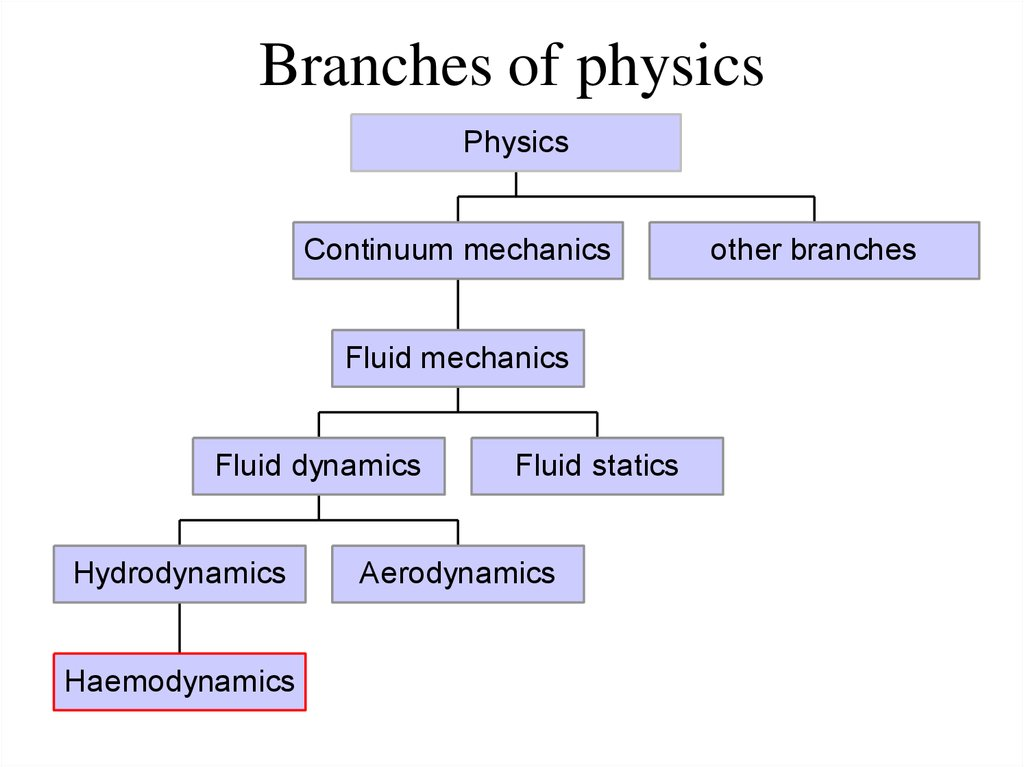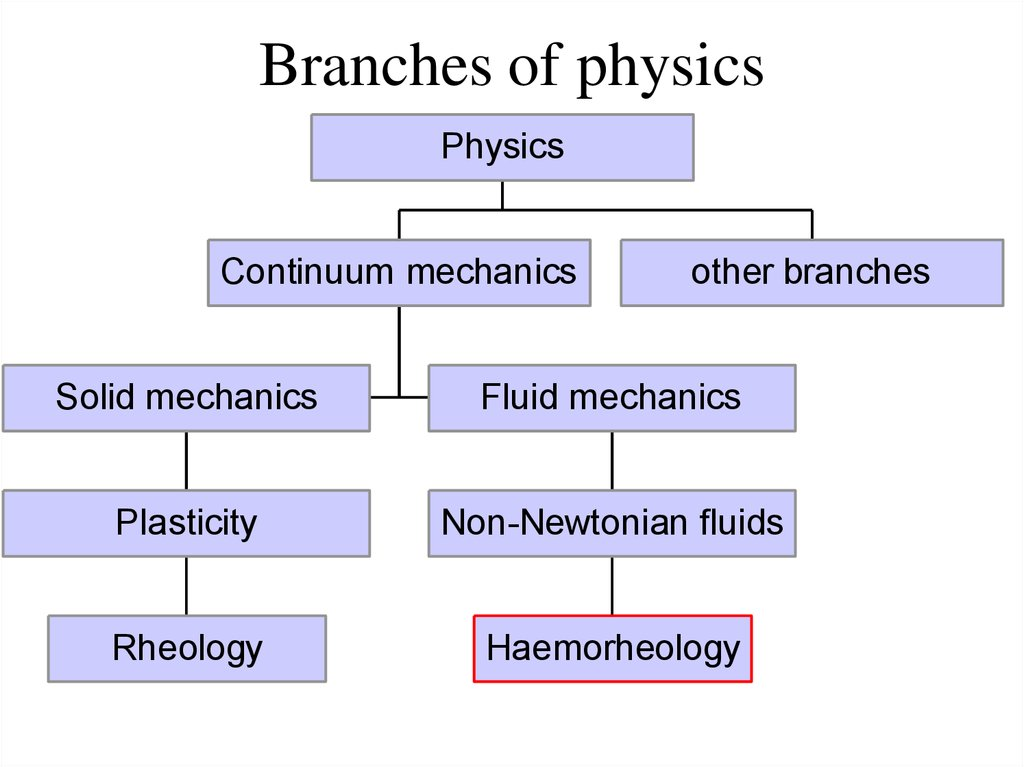# Haemodynamics Haemorheology

## 2. Branches of physics

Physics
Continuum mechanics
Fluid mechanics
Fluid dynamics
Hydrodynamics
Haemodynamics
Fluid statics
Aerodynamics
other branches

## 3. Branches of physics

Physics
Continuum mechanics
other branches
Solid mechanics
Fluid mechanics
Plasticity
Non-Newtonian fluids
Rheology
Haemorheology

## 4. Laminar and turbulent flow

(a) occurs when a fluid
flows in parallel
layers, with no
disruption between the
layers
(b) is a flow regime that
demonstrates chaotic
changes in pressure
and flow velocity

## 5. Viscosity

• The viscosity of a fluid is a measure of its
resistance to gradual deformation by shear
stress or tensile stress

## 6. Viscosity

Viscosity is a property of the fluid
which opposes the relative motion
between the two surfaces of the
fluid that are moving at different
velocities. When the fluid is
forced through a tube, the particles
which compose the fluid generally
move more quickly near the tube's
axis and more slowly near its
walls; therefore some stress is
needed to overcome the friction
between particle layers to keep the
fluid moving.

## 7. Newton's Law of Viscosity

dV
F
S
dZ
• F is the shear stress in the fluid
• η is a scalar constant of proportionality, the shear
viscosity of the fluid
• dV/dZ is the derivative of the velocity component
that is parallel to the direction of shear, relative to
displacement in the perpendicular direction.
• S is the surface (area) between fluid and the tube.

## 8.

• Non-Newtonian fluid
• Newtonian fluid
viscosity is dependent
fluid in which the
on shear rate or shear
viscous
stresses
rate history.
arising from its flow,
Shear thickening
at every point, are
(dilatant) - apparent
linearly proportional
viscosity
increases
to the local strain rate
with increased stress.
(the rate of change of
Shear
thinning
its deformation over
(pseudoplastic)
time).
apparent
viscosity
decreases
with
increased stress

## 9. Reynolds number

Is the important dimensionless quantity refers to ratio of
inertial forces to viscous forces within a fluid which is
subjected to relative internal movement due to different
fluid velocities, in which is known as a boundary layer in
the case of a bounding surface such as the interior of a
pipe.

## 10. Reynolds number

Used to help predict flow patterns in different fluid flow
situations.
At
low
Reynolds
numbers viscous forces
are dominant, and is
characterized by smooth,
constant fluid motion
(laminar flow).
At high Reynolds numbers
flow is dominated by inertial
forces, which tend to produce
chaotic eddies, vortices and
other
flow
instabilities
(turbulent flow).

## 11. Reynolds number

wherein:
vs - mean fluid velocity, [m/s]
L - characteristic length, [m]
μ - (absolute) dynamic fluid viscosity, [Pa*s]
ν - kinematic fluid viscosity: ν = μ / ρ, [m²/s]
ρ - fluid density, [kg*m-3]

## 12. Pascal's law

• Pascal's law is a principle in fluid
mechanics that states that a pressure change
occurring anywhere in a confined
incompressible
fluid
is
transmitted
throughout the fluid such that the same
change occurs everywhere

## 13. Bernoulli's principle

ρv2/2 + ρgh + p = const
• ρv2/2 is dynamic pressure,
• p = static pressure

## 14. Hagen–Poiseuille law

Q = ΔP πr4/8ηL
flow of liquid depends on following factors: like the
pressure gradient (∆P), the length of the narrow tube (L)
of radius (r) and the viscosity of the fluid (η) along with
relationship among them.
Assumptions:
•The tube is stiff, straight, and uniform
•Liquid is Newtonian , i.e., viscosity is constant
•The flow is laminar and steady, not pulsatile, and the velocity at the wall
is zero (no slip at the wall)

## 15. Hagen–Poiseuille law

Q = ΔPπr4 / 8ηL
The Pressure Gradient (∆P) : Shows the difference in the pressure between the two
ends of the tube, determined by the fact that any fluid will always flow from high
pressure to low pressure region and the flow rate is determined by the pressure
gradient (ΔP = P1 – P2)
Radius of tube: The liquid flow varies directly with the radius to the power 4.
Viscosity (η): The flow of the fluid varies inversely with the viscosity of the fluid and
as the viscosity of the fluid increases, the flow decreases vice versa.
Length of the Tube (L): The liquid flow is inversely proportional to the length of the
tube, therefore longer the tube, greater is the resistance to the flow.
Resistance(R): The resistance is described by 8ηL/πr4 and therefore the Poiseuille’s law
becomes
Q= (ΔP)/R

## 16. Cardiac output

CO = HR × SV
Is the volume of blood being pumped by the heart, in
particular by the left or right ventricle, per unit time.

## 17. Major factors influencing cardiac output

Cardiac Output
Stroke Vloume
Heart Rate
Heart size
Humoral regulation
(hormones)
Contractility
Autonomic innervation

## 18. Frank–Starling law

• The Frank–Starling law of the heart represents the
relationship between stroke volume and end diastolic
volume. The law states that the stroke volume of the
heart increases in response to an increase in the
volume of blood in the ventricles, before contraction
(the end diastolic volume), when all other factors
remain constant. As a larger volume of blood flows
into the ventricle, the blood stretches the cardiac
muscle fibers, leading to an increase in the force of
contraction.

## 19. Myocardial contractility

Myocardial contractility (cardiac inotropy) represents the innate
ability of the heart muscle to contract. Changes in the ability to
produce force during contraction result from incremental degrees of
binding between thick and thin filaments.
This results in better ejection of the blood in the ventricles.
Controlled by extrinsic factors
• sympathetic stimulation of the heart
• hormones
• K+ and Ca++ channel blockers

Preload is the end diastolic volume that
stretches the right or left ventricle of the
heart to its greatest dimensions under
variable physiologic demand. It is the initial
stretching of the cardiomyocytes prior to
contraction; therefore, it is related to the
sarcomere length at the end of diastole.

• Afterload is the stress in the wall of the left
ventricle during ejection. It is the end load
against which the heart contracts to eject
components: one factor is the aortic
pressure/ pulmonary pressure the left/right
ventricular muscle must overcome to eject
blood.

## 22. Vascular resistance

Vascular resistance is the resistance that
must be overcome to push blood through
the circulatory system and create flow.
Resistance is a factor of:
• Blood viscosity
• Total blood vessel length
• Vessel diameter

## 23. Rouleaux

Rouleaux are stacks or aggregations of red
blood cells which form because of the
unique discoid shape of the cells in
vertebrates. The flat surface of the discoid
RBCs gives them a large surface area to
make contact with and stick to each other;
thus forming a rouleau.

## 24. Rouleaux

Diameter of blood vessel is more than diameter of rouleaux

## 25. Rouleaux

Diameter of blood vessel is nearly equal to diameter of
rouleaux

## 26. Rouleaux

Diameter of blood vessel is less than diameter of RBC
1 KC
K
4
3
2,5 R
3

## 27. Hagen–Poiseuille law

Q = ΔPπr4 / 8ηL
Q= (ΔP)/R
Pressure gradient: created by the heart.
Resistance
Radius of tube: diameter of blood vessels.
Viscosity : property of blood.
Length of the tube

• 1.
• 2.
• 3.